## How to Count Cells that do not Contain Errors in Excel

This post will guide you how to count the number of cells that do not contain errors within a range of cells using a formula in Excel 2013/2016 or Excel office 365. How do I count the number of cells without any errors using a simple formula in Excel.

## 1. Count Number of Cells that do Not Contain Errors using Formula

You can use the SUMPRODUCT function in combining with NOT and ISERROR functions to count cells in a specified range of cells that do not contain errors

The below is a generic formula to count the number of cells that do not contain errors:

``=SUMPRODUCT(--NOT(ISERR(range)))``

Supposing that you wish to count the number of cells in range B1:B6 that do not contain errors, and you can use the following formula:

``=SUMPRODUCT(--NOT(ISERR(B1:B6)))``

LET’S SEE THAT HOW THIS FORMULA WORKS:

= NOT(ISERR(B1:B6))

The NOT and ISERROR functions can be used to identify the cells that do not contain an error. And it returns an array result with TRUE or FALSE values. The TRUE values indicate cells without errors, and FALSE values indicate cells with error values.

The array result is like this:

``{TRUE;TRUE;TRUE;FALSE;TRUE;FALSE}``

= –NOT(ISERR(B1:B6))

Then you still need to convert the above array result to their numeric values, 1 and 0, so that the SUMPRODUCT function can calculate the sum of products of corresponding numbers. And you can do this with a double negative operator. And the final array result is like this:

``{1;1;1;0;1;0}``

The SUMPRODUCT function sums the items in the above array and return the final result.

You can also use the SUM function to count the cell that do not contain errors in range B1:B6. And the generic formula is similar with the above formula. It need to be entered as an array formula through pressing `CTRL +SHIFT + Enter` to convert it as array formula. The formula looks like this:

``{=SUM(--NOT(ISERROR(B1:B6)))}``

## 2. Count Cells that do not Contain Errors using User Defined Function with VBA Code

If you want to count the number of cells that do not contain errors in a range, you can write a user defined function with VBA code. Here are the steps to create and run the function:

Step1: Press Alt + F11 keys to open the Microsoft Visual Basic for Applications window.

Step2: Click Insert > Module, and paste the following code in the Module Window.

```Function CountNonErrors_ExcelHow(rng As Range) As Long
Dim cell As Range
Dim count As Long
count = 0
For Each cell In rng
If Not IsError(cell.Value) Then
count = count + 1
End If
Next cell
CountNonErrors_ExcelHow = count
End Function```

Step3: Save and close the window.

Step4: In a blank cell, enter the formula:

``=CountNonErrors_ExcelHow(B1:B6)``

Where B1:B6 is the range you want to count.

Step5: Press Enter to get the result. This function will return the number of cells that do not contain errors in the specified range.

## 3. Video: Count Cells that do not Contain Errors in Excel

This video will show you how to count cells that do not contain errors in Excel using a simple formula and a VBA code.

## 4. Related Functions

• Excel SUMPRODUCT function
The Excel SUMPRODUCT function multiplies corresponding components in the given one or more arrays or ranges, and returns the sum of those products. The syntax of the SUMPRODUCT function is as below:= SUMPRODUCT (array1,[array2],…)…
• Excel ISERROR function
The Excel ISERROR function used to check for any error type that excel generates and it returns TRUE for any error type, and the ISERR function also can be checked for error values except #N/A error, it returns TRUE while the error is #N/A. The syntax of the ISERROR function is as below:= ISERROR (value)….
• Excel SUM function
The Excel SUM function will adds all numbers in a range of cells and returns the sum of these values. You can add individual values, cell references or ranges in excel.The syntax of the SUM function is as below:= SUM(number1,[number2],…)…
• Excel NOT function
The Excel NOT function returns the opposite of a given logical or Boolean value. For example, if you supplied with the value TRUE, the NOT function will return FALSE; If you supplied with the value FALSE, and the NOT function will TRUE. The syntax of the NOT function is as below:=NOT(logical)…

## How to Average and Ignore Errors in Excel

This post will guide you how to average a list of values and ignoring any errors in the given range in Excel 2013/2016 or Excel office 365. You can use the AVERAGEIF function or AGGREGATE function to calculate the average of a range of cells which might include some errors in Excel.

## Average Cells Ignoring Errors

Assuming you want to calculate the average of the cells that excluding any errors in range B1:B6, and you can use the following formula based on the AVERAGEIF function, like this:

=AVERAGEIF(B1:B6,”>10”)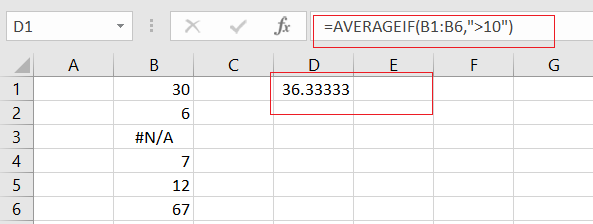Let’s See How This Formula Works:

The AVERAGEIF function can be used to calculate an average of some numeric values with one or more criteria. In the above example, the criteria is the expression of “>10”, and it means that you want to average all cell value in range B1:B6 which might include error values.

If you want to calculate the average of the cell values that ignoring any errors that might exist in the given range. And you can also use another array formula based on the AVERAGE function, the IF function and the ISERROR function. Like this:

=AVERAGE(IF(ISERROR(B1:B6),””,B1:B6))

Then you need to put this formula in a cell, and press Ctrl+Shift+Enter keys to make it as array formula. and you should see that the average result would be calculated.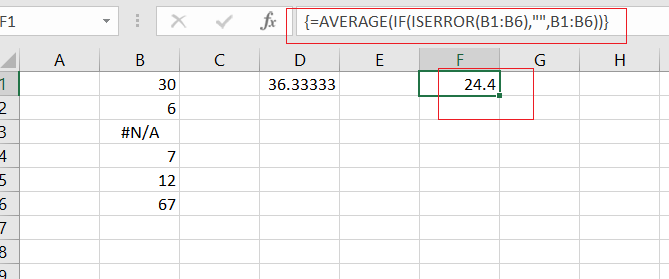### Related Functions

• Excel AVERAGE function
The Excel AVERAGE function returns the average of the numbers that you provided.The syntax of the AVERAGE function is as below:=AVERAGE (number1,[number2],…)….
• Excel AVERAGEIF function
The Excel AVERAGEAIF function returns the average of all numbers in a range of cells that meet a given criteria.The syntax of the AVERAGEIF function is as below:= AVERAGEIF (range, criteria, [average_range])….
• Excel IF function
The Excel IF function perform a logical test to return one value if the condition is TRUE and return another value if the condition is FALSE. The IF function is a build-in function in Microsoft Excel and it is categorized as a Logical Function.The syntax of the IF function is as below:= IF (condition, [true_value], [false_value])….
• Excel ISERROR function
The Excel ISERROR function used to check for any error type that excel generates and it returns TRUE for any error type, and the ISERR function also can be checked for error values except #N/A error, it returns TRUE while the error is #N/A. The syntax of the ISERROR function is as below:= ISERROR (value)….

## How to Count Number of Cells that Contain Errors in Excel

This post will guide you how to count the number of cells that contain errors within a range of cells using a formula in Excel 2013/2016.You can easily to count cells equal to a specific value or text string through COUNTIF function. But if there is an easy way to count cells contain errors in your data range.

## Count Number of Cells that Contain Errors

You should know that there are several types of errors such as: `#N/A`, `#REF!` or `#VALUE!` In Excel. And you only want to count the number of all types of errors with a formula in Excel. This tutorial will show you how to count the number of cells in a specified range of cell A1:B6 that contain errors using an Excel formula ,with the SUMRODUCT and ISERROR functions. Like below:

=SUMPRODUCT(–ISERROR(A1:B6))

###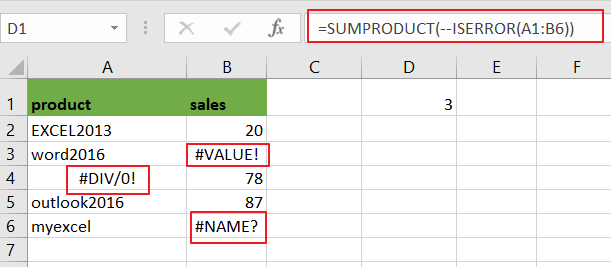Note: A1:B6 is the range from which to count the cells that contain errors.

LET’S SEE THAT HOW THIS FORMULA WORKS:

The SUMPRODUCT function can be used to multiply the corresponding components in the given arrays. If there is only one array, SUMPRODUCT function would return the sum of values in the array.

=ISERROR(A1:B6)

###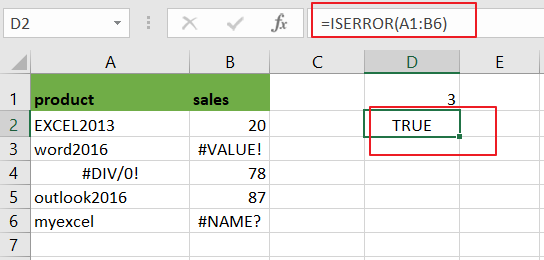The ISERROR function is used to identify the cells that contain an error. And returns True if cell contain any error values. In this case, ISERROR function returns an array that contain TRUE or FALSE values, like this:

={FALSE,FALSE;FALSE,FALSE;FALSE,TRUE;TRUE,FALSE;FALSE,FALSE;FALSE,TRUE}

=–ISERROR(A1:B6)

###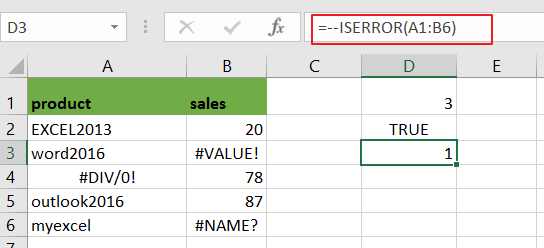You still need to convert the TRUE or FALSE values to number 1 and 0 by using a double negative operator. And the result array looks like this:

{0,0;0,0;0,1;1,0;0,0;0,1}

###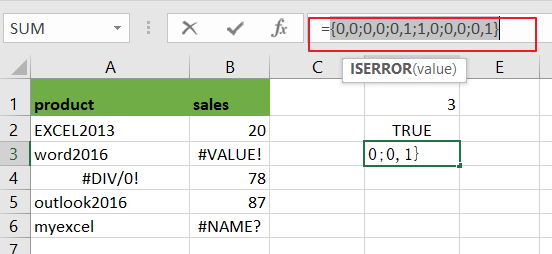The SUMPRODUCT function would add the value of the above array and return the final result.

You can also use another array formula based on the SUM function, IF function and ISERROR function to count the number of all types of errors in a range in Excel. Like this:

{=SUM(IF(ISERROR(A1:B6),1))}

###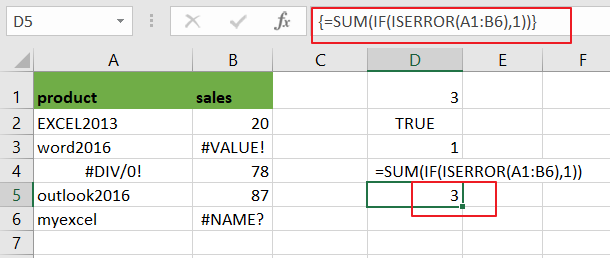Note: you need to press CTRL + SHIFT + ENTER short keys to covert its as a array formula.

### Related Functions

• Excel SUMPRODUCT function
The Excel SUMPRODUCT function multiplies corresponding components in the given one or more arrays or ranges, and returns the sum of those products. The syntax of the SUMPRODUCT function is as below:= SUMPRODUCT (array1,[array2],…)…
• Excel COUNTIF function
The Excel COUNTIF function will count the number of cells in a range that meet a given criteria. This function can be used to count the different kinds of cells with number, date, text values, blank, non-blanks, or containing specific characters.etc.= COUNTIF (range, criteria)…
• Excel IF function
The Excel IF function perform a logical test to return one value if the condition is TRUE and return another value if the condition is FALSE. The IF function is a build-in function in Microsoft Excel and it is categorized as a Logical Function.The syntax of the IF function is as below:= IF (condition, [true_value], [false_value])….
• Excel ISERROR function
The Excel ISERROR function used to check for any error type that excel generates and it returns TRUE for any error type, and the ISERR function also can be checked for error values except #N/A error, it returns TRUE while the error is #N/A. The syntax of the ISERROR function is as below:= ISERROR (value)….
• Excel SUM function
The Excel SUM function will adds all numbers in a range of cells and returns the sum of these values. You can add individual values, cell references or ranges in excel.The syntax of the SUM function is as below:= SUM(number1,[number2],…)…

## How to Compare Two Columns and Remove the Duplicate Values by Formula in Excel

To compare two columns and remove the duplicate values, we have already posted a tutorial about how to solve this problem by ‘Conditional Formatting’ feature ‘Remove Duplicate’ rule. Actually, there are some other ways to solve it as well. If you are not familiar with ‘Conditional Formatting’ feature, you can also use a formula to compare columns and remove the duplicate values. In this article, we will introduce you how to apply IF, ISERROR and MATCH functions in a formula to compare data, I hope after reading this article, you will find the way to resolve your problem.

Precondition:

See screenshot below. We prepare two lists with fruits. As the two lists are simple, so we can see ‘Apple’ exists in both two columns obviously. But if the list is very long, we cannot compare them easily, we need to use formula to compare them.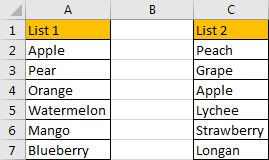## Method: Compare Two Columns and Remove Duplicates by Formula

Step 1: In B2 which is just between two columns, enter the formula =IF(ISERROR(MATCH(A2,\$C\$2:\$C\$7,0)),”Not Duplicate”,”Duplicate”).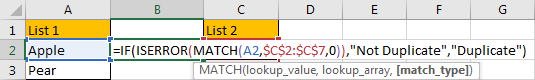Step 2: Press Enter to get value. Verify that we get ‘Duplicate’ in B2. That means ‘Apple’ in list1 also exists in list2.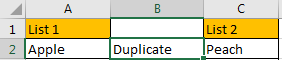Step 3: Drag the fill handle down till the end of the list.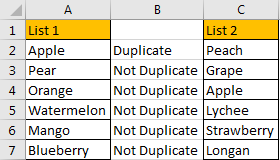Step 4: Now we already find out duplicate values, if you want to remove the duplicate value from list1, you can click B1, then click Data->Filter under Sort & Filter group.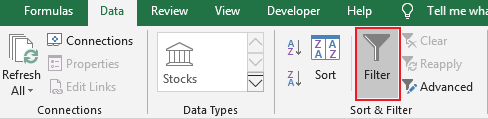The filter dropdown list is created.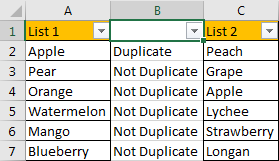Step 5: Click the small arrow button to load criteria, check on ‘Duplicate’, then click OK.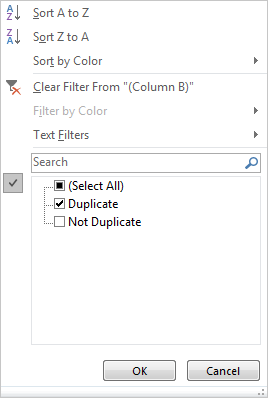Duplicate value in list1 is displayed.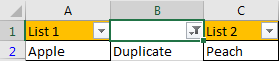Step 6: Just remove duplicate value from filtered list1. Now there is no duplicate value now.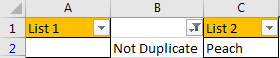### Related Functions

• Excel IF function
The Excel IF function perform a logical test to return one value if the condition is TRUE and return another value if the condition is FALSE. The IF function is a build-in function in Microsoft Excel and it is categorized as a Logical Function.The syntax of the IF function is as below:= IF (condition, [true_value], [false_value])….
• Excel ISERROR function
The Excel ISERROR function used to check for any error type that excel generates and it returns TRUE for any error type, and the ISERR function also can be checked for error values except #N/A error, it returns TRUE while the error is #N/A. The syntax of the ISERROR function is as below:= ISERROR (value)….
• Excel MATCH function
The Excel MATCH function search a value in an array and returns the position of that item.The syntax of the MATCH function is as below:= MATCH  (lookup_value, lookup_array, [match_type])….

## How to Compare Two Columns to Find Missing Value (Unique Value) in Excel

Suppose we have two lists, list A contains all students, list B only contains students who passed exam. So, list A is longer than list B, and students in list B are all included in list A. If we want to find out which student failed the exam, we can though compare the two list and find out the missing value to confirm the name list. Actually, in our work we often meet the situations that we need to compare two lists and find out the missing values. In this tutorial, we will help you to find out missing values via two ways, the first one is by Conditional Formatting function in excel, the second one is by using formula with VLOOKUP function.

Precondition:

Prepare two lists. List A contains all students. List B contains partial of them.

## Method 1: Compare Two Columns to Find Missing Value by Conditional Formatting

Step 1: Select List A and List B.

Step 2: Click Home in ribbon, click Conditional Formatting in Styles group.

Step 3: In Conditional Formatting dropdown list, select Highlight Cells Rules->Duplicate Values.

Step 4: In Duplicate Values dialog, select Unique in dropdown list. Keep default value in values with dropdown list. Then click OK.

Step 5: Verify that unique values are marked with dark red properly.

This way can be used in finding unique values from two lists.

## Method 2: Compare Two Columns to Find Missing Value by Formula

Insert a new column between List A and List B.

Step 1: In B2 enter the formula =ISERROR(VLOOKUP(A2,\$C\$2:\$C\$11,1,FALSE)). VLOOLIP function can help us to look up match value from List B. ISERROR function is used for checking whether match value exists or not, it returns TRUE or FALSE.

Step 2: Drag the fill handle down. Verify that B4 and B10 are filled with TRUE.

So, in List A, Calvin and Peter are students who failed in exam.

Step 3: If you want to show student name in new list directly, you can update formula =IF(ISERROR(VLOOKUP(A2,\$C\$2:\$C\$11,1,FALSE)),A2, “”).

Step 4: After applying above formula for the following cells, missing value will display in new list directly.

## Video: Compare Two Columns to Find Missing Value (Unique Value) in Excel

### Related Functions

• Excel IF function
The Excel IF function perform a logical test to return one value if the condition is TRUE and return another value if the condition is FALSE. The IF function is a build-in function in Microsoft Excel and it is categorized as a Logical Function.The syntax of the IF function is as below:= IF (condition, [true_value], [false_value])….
• Excel VLOOKUP function
The Excel VLOOKUP function lookup a value in the first column of the table and return the value in the same row based on index_num position.The syntax of the VLOOKUP function is as below:= VLOOKUP (lookup_value, table_array, column_index_num,[range_lookup])….
• Excel ISERROR function
The Excel ISERROR function used to check for any error type that excel generates and it returns TRUE for any error type, and the ISERR function also can be checked for error values except #N/A error, it returns TRUE while the error is #N/A. The syntax of the ISERROR function is as below:= ISERROR (value)….

## How to Ignore Error Values When Calculating the Average in Excel

This post will guide you how to ignore error values when getting average for a range of cells in Excel. How do I average a range of cells ignoring error values, such as: #div/0!.

Assuming that you have a list of range of cells that you want to calculate the average of those cells which including error values , such as: #div/0 or #NAME?, etc. When you use the Average function to calculate those cells, you will get an error result. So How to handle this in Excel.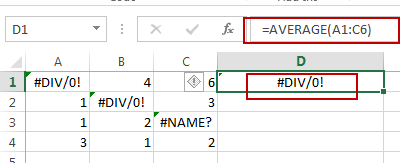If you want to ignoring any errors that might exist in the given range, you can use AVERAGEIF function or AVERAGE function with IF function to achieve the result. Like this:

`=AVERAGEIF(A1:C4,”>=0”)`

Or

`=AVERAGE(IF(ISERROR(A1:C4),””,A1:C4))`

OR

`=AVERAGE(IF(ISNUMBER(A1:C4),A1:C4))`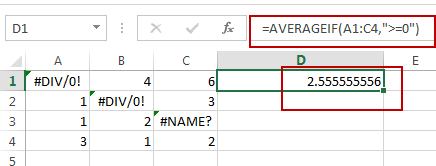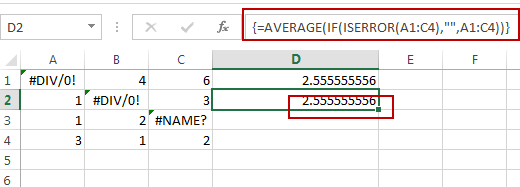Note: The second and third formula need to press Ctrl + Shift +  Enter keys, as those formulas are Array Formula. A1:C4 is the data range that you wish to average. And you need to change it as you need.

Let’s see how the first formula works: the AVERAGEIF function can be used to calculate an average of numeric values with one or more criteria for a given range. And In this example, the criteria is the expression “>=0”, it will filter out all error values, and just to calculate the positive numeric values in the given range.

### Related Functions

• Excel IF function
The Excel IF function perform a logical test to return one value if the condition is TRUE and return another value if the condition is FALSE. The IF function is a build-in function in Microsoft Excel and it is categorized as a Logical Function.The syntax of the IF function is as below:= IF (condition, [true_value], [false_value])….
• Excel AVERAGE function
The Excel AVERAGE function returns the average of the numbers that you provided.The syntax of the AVERAGE function is as below:=AVERAGE (number1,[number2],…)….
• Excel AVERAGEIF function
The Excel AVERAGEAIF function returns the average of all numbers in a range of cells that meet a given criteria.The syntax of the AVERAGEIF function is as below:= AVERAGEIF (range, criteria, [average_range])….
• Excel IFERROR function
The Excel IFERROR function returns an alternate value you specify if a formula results in an error, or returns the result of the formula.The syntax of the IFERROR function is as below:= IFERROR (value, value_if_error)….
• Excel ISNUMBER function
The Excel ISNUMBER function returns TRUE if the value in a cell is a numeric value, otherwise it will return FALSE.The syntax of the ISNUMBER function is as below:= ISNUMBER (value)…

## How to Compare Two Lists in Different Worksheets using VLOOKUP in Excel

This post will guide you how to compare two lists in different worksheets in Excel. How do I compare two lists in different worksheets with VLOOKUP function in Excel 2013/2016.

## Comparing Two Lists in Different Worksheets

Assuming that you have two lists of data in the different worksheets, and you want to compare those two lists and try to check the values in the first list(Sheet2 – A1:A5) if it is in another list (Sheet6 – A1:A5). How to do it. You can use an Excel formula based on the VLOOKUP function, the IF function and the ISERROR function.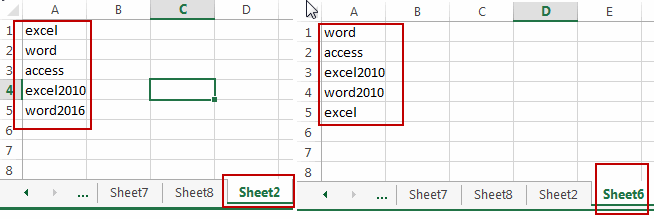Here is the formula that comparing two lists in different worksheets:

`= IF(ISERROR(VLOOKUP(A1,'Sheet6'!\$A\$1:\$A\$5,1,0)),"No","Yes")`

You need to type this formula into cell B1 in the first worksheet that containing the first list data. And then drag the Fill Handle down to the cells to comparing other values with the second list in another worksheet.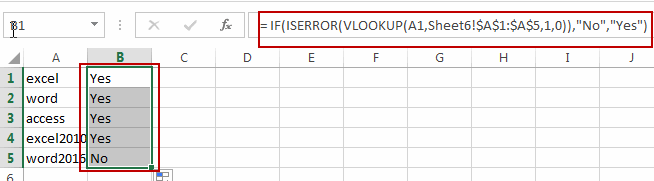You would notice that the checking results have been returned in column B. if it returns Yes, it indicates that this cell value is also in the second list in another worksheet. If it return No, it indicates that this cell value is not in the second list.

### Related Functions

• Excel IF function
The Excel IF function perform a logical test to return one value if the condition is TRUE and return another value if the condition is FALSE. The IF function is a build-in function in Microsoft Excel and it is categorized as a Logical Function.The syntax of the IF function is as below:= IF (condition, [true_value], [false_value])….
• Excel VLOOKUP function
The Excel VLOOKUP function lookup a value in the first column of the table and return the value in the same row based on index_num position.The syntax of the VLOOKUP function is as below:= VLOOKUP (lookup_value, table_array, column_index_num,[range_lookup])….
• Excel IFERROR function
The Excel IFERROR function returns an alternate value you specify if a formula results in an error, or returns the result of the formula.The syntax of the IFERROR function is as below:= IFERROR (value, value_if_error)….

## How to Sum Range Ignoring Errors in Excel

This post will guide you how to sum range of cells ignoring Errors in Excel. How do I sum and ignore errors with a formula in Excel. How to sum range ignoring all error values with VBA macro in Excel.

## Sum Range Ignoring Errors with Formula

Assuming that you have a list of data in range A1:C4, in which contain numeric values and the error values, such as: #NAME?, #DIV/0, etc. and you want to sum this range but ignoring all error values. How to do it. You can use an Excel Array formula based on the SUM function, the IF function and the ISERROR function to achieve the result. Like this:

`=SUM(IF(ISERROR(A1:C4),””,A1:C4))`

You need to type this formula into a blank cell and press Ctrl + Shift + Enter keys on your keyboard to change this formula as array formula.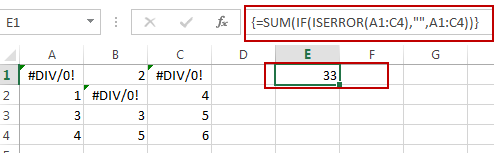## Sum Range Ignoring Errors with VBA

You can also use an Excel VBA Macro to achieve the same result of summing a selected range of cells ignoring all error values. Here are the steps:

#1 open your excel workbook and then click on “Visual Basic” command under DEVELOPER Tab, or just press “ALT+F11” shortcut.#2 then the “Visual Basic Editor” window will appear.

#3 click “Insert” ->”Module” to create a new module.#4 paste the below VBA code  into the code window. Then clicking “Save” button.

```Sub SumRangeIgnoringError()

Set myRange = Application.Selection
Set myRange = Application.InputBox("Select one Range:", "SumRangeIgnoringError", myRange.Address, Type:=8)
For Each myCell In myRange
If Not (IsError(myCell.Value)) Then
lSum = lSum + myCell.Value
End If
Next
MsgBox lSum, , "SumRangeIgnoringError"
End Sub```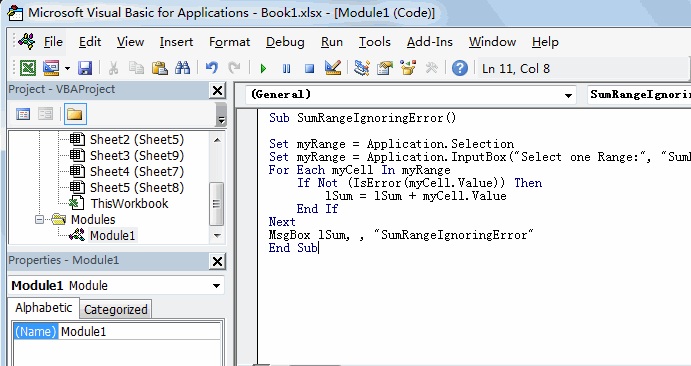#5 back to the current worksheet, then run the above excel macro. Click Run button.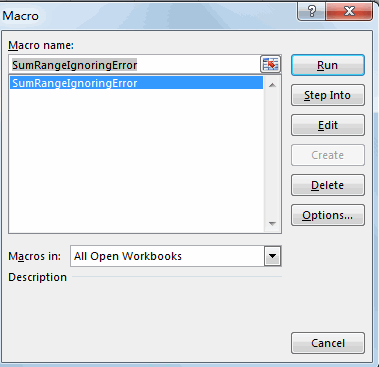#6 Please select one range that contain error values. Click OK button.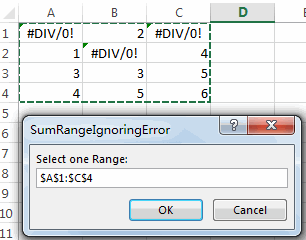#7 Let’s see the result: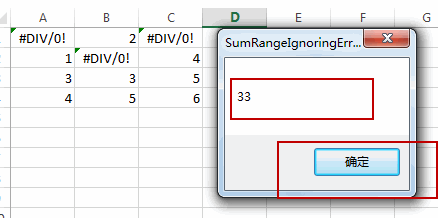### Related Functions

• Excel SUM function
The Excel SUM function will adds all numbers in a range of cells and returns the sum of these values. You can add individual values, cell references or ranges in excel.The syntax of the SUM function is as below:= SUM(number1,[number2],…)…
• Excel IF function
The Excel IF function perform a logical test to return one value if the condition is TRUE and return another value if the condition is FALSE. The IF function is a build-in function in Microsoft Excel and it is categorized as a Logical Function.The syntax of the IF function is as below:= IF (condition, [true_value], [false_value])….
• Excel ISERROR function
The Excel ISERROR function used to check for any error type that excel generates and it returns TRUE for any error type, and the ISERR function also can be checked for error values except #N/A error, it returns TRUE while the error is #N/A. The syntax of the ISERROR function is as below:= ISERROR (value)….

## Check If Value in a Column exists in Another Column in Excel

This post will guide you how to check if one value in a column exists in another column in Excel. How do I check if value in column A exists in Column B with a formula in Excel. How to determine if values exists in another column in Excel.

## Check If Value in a Column exists in Another Column

Assuming that you have a list of data in two columns in range A1:B5, and you want to check if one value in column A exists in column B, if True, then return true, otherwise, returns False. How to achieve it. You can create a new formula based on the IF function, the ISERROR function and the VLOOKUP function. Like this:

=IF(ISERROR(VLOOKUP(A1,\$B\$1:\$B\$5, 1, FALSE)),FALSE,TRUE )

Type this formula into cell C1, and press Enter key in your keyboard, and then drag the autofill handle over other cells to apply this formula to check other cells in Column A.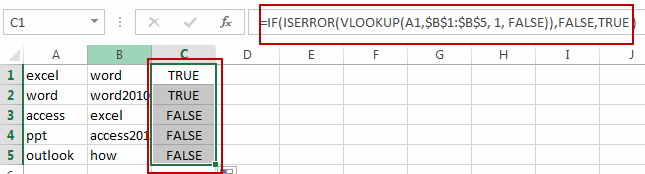### Related Functions

• Excel IF function
The Excel IF function perform a logical test to return one value if the condition is TRUE and return another value if the condition is FALSE. The IF function is a build-in function in Microsoft Excel and it is categorized as a Logical Function.The syntax of the IF function is as below:= IF (condition, [true_value], [false_value])….
• Excel VLOOKUP function
The Excel VLOOKUP function lookup a value in the first column of the table and return the value in the same row based on index_num position.The syntax of the VLOOKUP function is as below:= VLOOKUP (lookup_value, table_array, column_index_num,[range_lookup])….
• Excel ISERROR function
The Excel ISERROR function used to check for any error type that excel generates and it returns TRUE for any error type, and the ISERR function also can be checked for error values except #N/A error, it returns TRUE while the error is #N/A. The syntax of the ISERROR function is as below:= ISERROR (value)….

## Find Duplicate Values in Two Columns

This tutorial will show you how to find duplicate values in two given columns in Excel. Or How to compare two columns and find duplicate entries in Microsoft Excel worksheet. And you will learn two methods to compare columns so that the duplicate values can be highlighted or listed in a range of Cells.

Assuming that you need to compare two columns (Column A and Column B) to get the duplicate values in your worksheet, you can find duplicate values in two columns with Excel Formula, or Excel VBA Macro code.

## Method 1: Find duplicate values in two columns with Excel Formula

To compare two given columns and find duplicate values in Excel, you can use a combination of the IF function, the ISERROR function, and the MATCH function to create an Excel formula. So you can use the following formula:

`=IF(ISERROR(MATCH(A1,\$B\$1:\$B\$4,0))," ",A1)`

Now you need to type this formula in Cell C1, press Enter key, drag AutoFill Handle down to Cell C2:C4, you will see all of the duplicated values are displayed in Column C.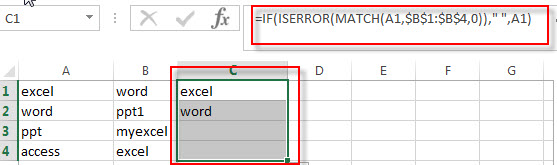## Method 2: Find duplicate values in two columns with VBA Macro code

If you are familiar with the programming language and you can use a Visual Basic Macro to compare the value in two columns and then find duplicate values, just refer to the following steps:

1# click on “Visual Basic” command under DEVELOPER Tab.2# then the “Visual Basic Editor” window will appear.

3# click “Insert” ->”Module” to create a new module4# paste the below VBA code into the code window. Then clicking “Save” button.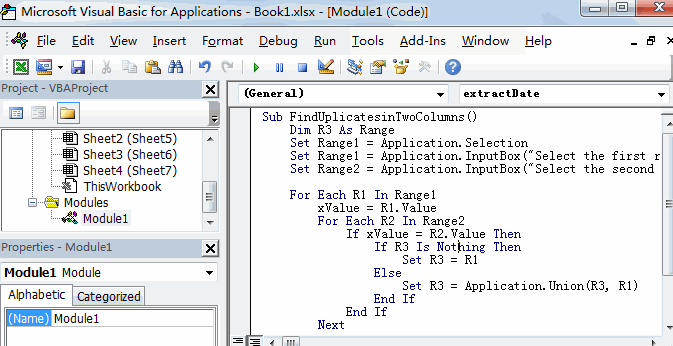```Sub FindUplicatesinTwoColumns()
Set Range1 = Application.Selection
Set Range1 = Application.InputBox("Select the first range in one column:", "FindUplicatesinTwoColumns", Range1.Address, Type:=8)
Set Range2 = Application.InputBox("Select the second range in another column:", "FindUplicatesinTwoColumns", Type:=8)

For Each R1 In Range1
xValue = R1.Value
For Each R2 In Range2
If xValue = R2.Value Then
If R3 Is Nothing Then
Set R3 = R1
Else
Set R3 = Application.Union(R3, R1)
End If
End If
Next
Next
R3.Interior.ColorIndex=3

End Sub```

5# back to the current worksheet, then run the above excel macro.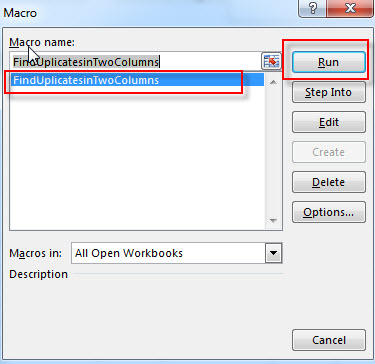6# Select the first range A1:A4 in Column A, click OK button, then select the second range B1:B4 in Column B, click OK button.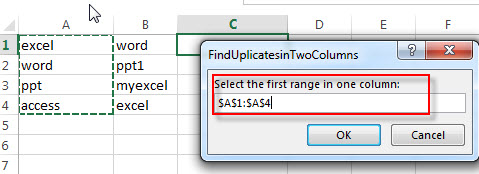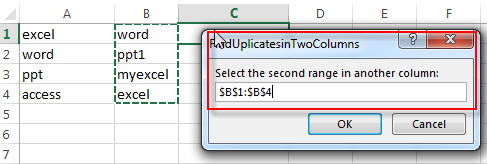7# let’s see the result: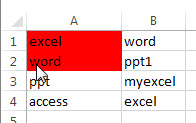## Method 3: Find duplicate values in two columns with Conditional Formatting feature

You can use conditional formatting with on a formula based on the COUNTIF function and the AND function to find the duplicate values in two specified columns and then highlighted them. Just do it following:

1# Select the entire Column A via click on the Column Header and then the column A will be highlighted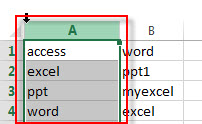2# on the HOME tab, click the Conditional Formatting command under Styles group. Then select New Rules… from the drop-down menu list.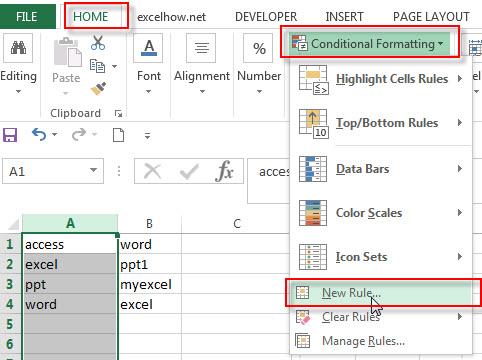3# select Use a formula to determine which cells to format as Rule Type in the New Formatting Rule window

4# Type the following formula in the Format values where this formula is true: box

`=COUNTIf(\$B:\$B, \$A1)`

5# click the Format… button, then the Format Cells window will appear.

6# in the “Format Cells” window, switch to the Fill tab, choose the background color, and then click OK button.

7#you will be back to the New Formatting Rule windows and you can check a preview of the formatting you have selected. Then click OK button.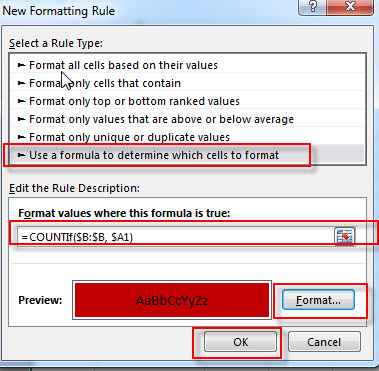Then the conditional formatting rule will be applied to all values in two columns and highlighted the duplicate values.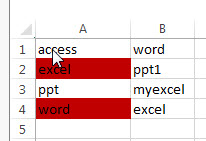### Related Functions

• Excel IF function
The Excel IF function perform a logical test to return one value if the condition is TRUE and return another value if the condition is FALSE. The IF function is a build-in function in Microsoft Excel and it is categorized as a Logical Function.The syntax of the IF function is as below:= IF (condition, [true_value], [false_value])….
• Excel ISERROR function
The Excel ISERROR function used to check for any error type that excel generates and it returns TRUE for any error type, and the ISERR function also can be checked for error values except #N/A error, it returns TRUE while the error is #N/A. The syntax of the ISERROR function is as below:= ISERROR (value)….
• Excel MATCH function
The Excel MATCH function search a value in an array and returns the position of that item.The syntax of the MATCH function is as below:= MATCH  (lookup_value, lookup_array, [match_type])….
• Excel COUNTIF function
The Excel COUNTIF function will count the number of cells in a range that meet a given criteria. This function can be used to count the different kinds of cells with number, date, text values, blank, non-blanks, or containing specific characters.etc.= COUNTIF (range, criteria)…
• Excel AND function
The Excel AND function returns TRUE if all of arguments are TRUE, and it returns FALSE if any of arguments are FALSE.The syntax of the AND function is as below:= AND (condition1,[condition2],…)…

### Related Posts

• Highlight Rows
You will learn that how to change the color of the entire rows if the value of cells in a specified column meets your conditions, such as, if the value of cells is equal to or greater than a certain number or text values, then excel should be highlight entire rows or change a row color as you need.…
• Find Duplicate Rows
If you want to check the entire row that duplicated or not, if True, then returns “duplicates” value, otherwise, returns “no duplicates”. You can create a formula based on the IF function and the SUMPRODUCT function..…
• Highlight Duplicate Rows
this post will talk that how to highlight entire rows that are duplicates in excel 2016, 2013 or lower version. Or how to change the background color of duplicate rows..…
• Highlight duplicate values
this post will teach you how to highlight duplicate values in the range of cells in excel. Normally, you may be need to identify duplicate values with a range of cells in Excel. And there is one of the fasted way that is using conditional formatting feature in Microsoft Excel……

## Excel ISError Function

This post will guide you how to use Excel ISERROR function with syntax and examples in Microsoft excel.

## Description

The Excel ISERROR function used to check for any error type that excel generates and it returns TRUE for any error type, such as: #N/A, #VALUE!,#REF!,#DIV0!, #NAME?, etc. and the ISERR function also can be checked for error values except #N/A error, it returns TRUE while the error is #N/A.

The ISERROR function is a build-in function in Microsoft Excel and it is categorized as an Information Function.

The ISERROR function is available in Excel 2016, Excel 2013, Excel 2010, Excel 2007, Excel 2003, Excel XP, Excel 2000, Excel 2011 for Mac.

## Syntax

The syntax of the ISERROR function is as below:

`= ISERROR (value)`

Where the ISERROR function argument is:

• Value -This is a required argument. The value that you want to test.

Example

The below examples will show you how to use Excel ISERROR Function to test if the value is any of error values. If so, return TRUE.

#1 using the following formula to check #N/A error.

`= ISERROR (B1)`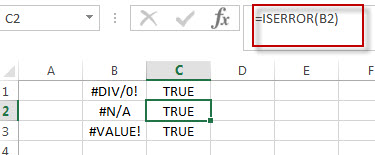#2 using the ISERROR function to check Cell B1 contains the value “excel”, enter the below formula in Cell C1 formula box:

`=ISERROR(B1)`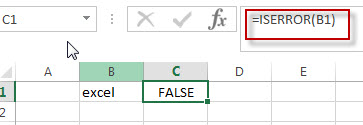As it is not an error type, so returns FALSE.

### Related Functions

• Excel ISNUMBER Function
The Excel ISNUMBER function returns TRUE if the value in a cell is a numeric value, otherwise it will return FALSE.The syntax of the ISNUMBER function is as below:=ISNUMBER (value)
• Excel ISERR Function
The Excel ISERR function returns TRUE if the value is any error value except #N/A.The syntax of the ISERR function is as below:= ISERR (value)…
• Excel ISBlank Function
The Excel ISBLANK function returns TRUE if the value is blank or null.The syntax of the ISBLANK function is as below:=ISBLANK (value)…
• Excel ISNA Function
The Excel ISNA function used to check if a cell contains the #N/A error, if so, returns TRUE; otherwise, the ISNA function returns FALSE. The syntax of the ISNA function is as below:=ISNA(value)…
• Excel ISTEXT Function
The Excel ISTEXT function used to check if a value is text. If so, returns TRUE; if the text is not text, the function will return FALSE. The syntax of the ISTEXT function is as below:=ISTEXT(value)…
• Excel ISNONTEXT Function
The Excel ISNONTEXT function used to check if a value is text. If so, returns FALSE; if the text is not text, the function will return TRUE. The syntax of the ISNONTEXT function is as below:=ISNONTEXT (value)…
• Excel ISREF Function
The Excel ISREF function used to check if a value is a valid reference. If so, returns TRUE; if the value is not a reference, the function will return FALSE. The syntax of the ISREF function is as below:=ISREF(value)…